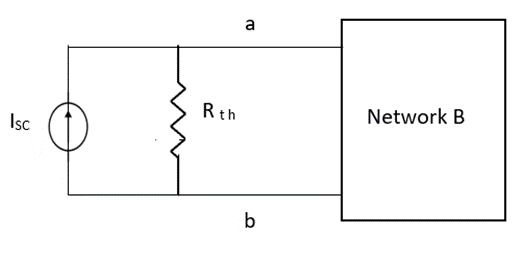Goseeko blog# What is Norton’s Theorem?

Norton’s Theorem states that a two-terminal network containing sources and passive elements is equivalent to an independent current source in parallel with the network impedance with all independent sources removed. The current of the current source has the same magnitude and reference direction as that of the current which would exist at the terminal in the original network if the terminals were short-circuited.

The short circuit current is then given as

Isc = Vth/Rth

Norton’s equivalent is obtained by source conversion of Thevenin’s equivalent circuit.## CONDITIONS FOR APPLICATION OF NORTON’S THEOREM

For network A:

1. Network A should contain linear elements.

2. Network A can have independent and dependent current and voltage sources.

3. If network A has a dependent source then the controlling parameter must lie in network A itself.

4. Network A should not have any source coupling and magnetic coupling.

o   For network B:

1. It can have linear and non-linear elements.

2. It can have dependent and independent voltage and current sources.

3. It should not have any source and magnetic coupling with network A.

Method for finding Rth:

Firstly, open circuit terminal A and B.

1. If the network is operating with only independent sources:

a. Make all sources zero in network A.

b. Find out the equivalent resistance across terminals A and B.

2. If network A is operating with independent and dependent sources:

a. Make all independent sources zero in network A.

b. Connect a generation between A and B.

3. If the network is operating with only dependent sources:

Connect generation between A and B

Method for Vth:

First, open circuit terminal A and B.

Find out the voltage between A and B this is Vth

Method for Isc:

1. Isc =Vth/Rth

2. Remove network B and S.C. the terminal A and B and current from terminal A to B Isc.

## Examples

Question: Find Vth and Rth for the circuit shown below?

Solution

Finding the Thevenin’s Resistance Rth

The Rth   is calculated from the terminal a and b. For that we remove all the sources present in the bilateral network. The voltage source is shorted. The circuit after changes is

Then the value of Resistance Rth is

The value of voltage Vth is

Finding Isc from circuit directly:

Applying KCL to the above circuit we get